## Chapter 2 Polynomials R.D. Sharma Solutions for Class 10th Math Exercise 2.3

Exercise 2.3

1. Apply division algorithm to find the quotient q(x) and remainder r(x) on dividing f(x) by g(x) in each of the following :
(i) f(x) = x3 - 6x2 + 11x - 6, g(x) = x2 + x + 1
(ii) f(x) 10x4 + 17x3 - 62x2 + 30x - 105(x) = 2x2 + 7x + 1
(iii) f(x) = 4x3 + 8x2 + 8x + 7:9(x) = 2x2 - x + 1
(iv) f(x) = 15x3 - 20x2 + 13x - 12; g(x) = x2 - 2x + 2

Solution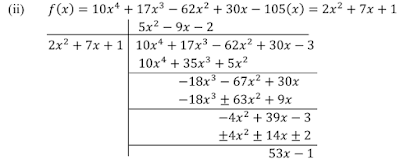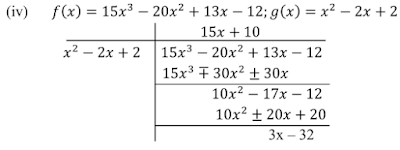2. Check whether the first polynomial is a factor of the second polynomial by applying the division algorithm:
(i) g(t) = t2 - 3; f(t) = 2t4 + 3t3 - 2t2 - 9t

(ii) g(x) = x2 - 3x + 1, f(x) = x5 - 4x3 + x2 + 3x + 1
(iii) g(x) = 2x2 - x + 3, f(x) = 6x5 - x4 + 4x3 - 5x2 - x - 15

Solution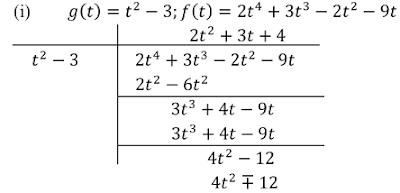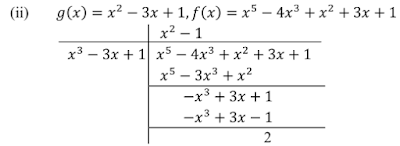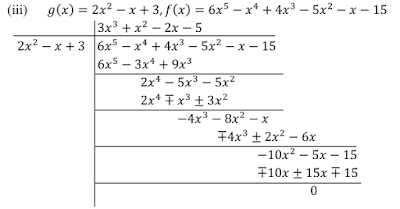3. Obtain all zeros of the polynomial f(x) = 2x4 + x3 - 14x2 - 19x - 6, if two of its zeors are -2 and -1.

Solution4. Obtain all zeros of f(x) = x3 + 13x2 + 32x + 20, if one of its zeros is -2.

Solution5. Obtain all zeros of the polynomial f(x) = x4 - 3x2 = x2 + 9x - 6 if two of its zeros are -√3, and √3 .

Solution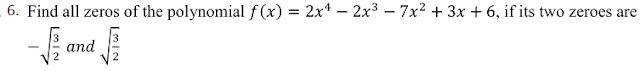Solution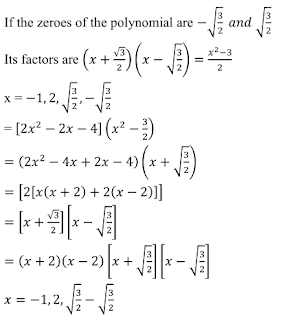7. Find all the zeroes of the polynomial x4 + x3 - 34x2 - 4x + 120, if two of its zeroes are 2 and -2 .

Solution8. Find all zeros of the polynomial 2x4 + 7x3 - 19x2 - 14x + 30, if two of its zeros are √2 and -√2 .

Solution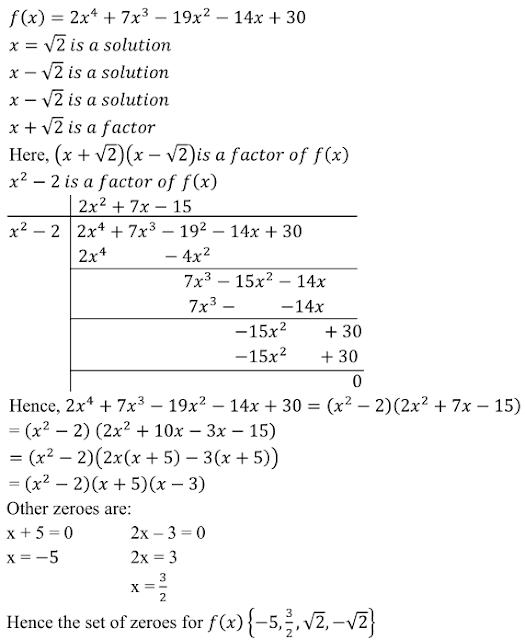9. Find all the zeros of the polynomial 2x3 + x2 - 6x - 3, if two of its zeros are -√3 and √3.

Solution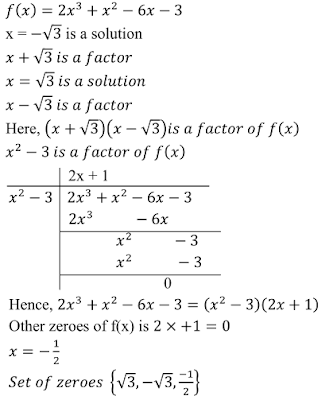10. Find all the zeros of the polynomial x3 + 3x2 - 2x - 6, if two of its zeros are -√2 and √2.

Solution11. What must be added to the polynomial f(x) = x4 + 2x3 - 2x2 + x -1 so that the resulting polynomial is exactly by x2 + 2x - 3 ?

Solution12. What must be subtracted from the polynomial f(x) = x4 + 2x3 - 13x2 - 12x + 21 so that the resulting polynomial is exactly divisible by x2 - 4x + 3 ?

Solution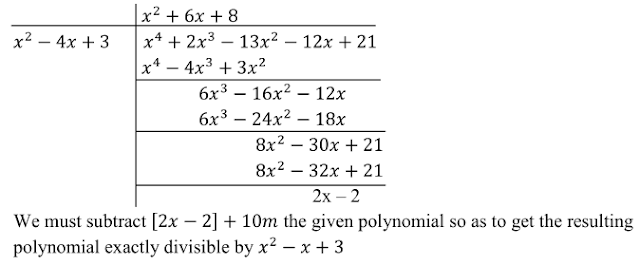13. Given that √2 is a zero of the cumbic polynomial 6x3 + √2x2 – 10x - 4√2 , find its other two zeroes.
Solution

We know that if x = a is a zero of a polynomial, and then x -1 is a factor of f (x).
It is given that √2 is a zero of the cubic polynomial f (x) = 6x3 + √2x2 – 10x - 4√2 .
Therefore, x - √2 is a factor of f(x) . Now, we divide 6x3 + √2x2 – 10x - 4√2 by x - √2 to find the other zeroes of f(x).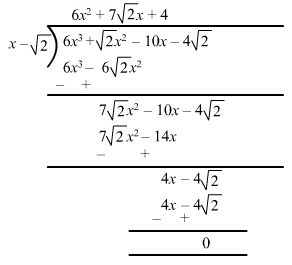∴ Quotient = 6x2 + 7√2x + 4 and remainder = 0 .
By using division algorithm , we have f(x) = g(x) × q(x) + r(x) .
f(x) = (x - √2) (6x2 + 7√2x + 4) + 0
= (x - √2) (√2x + 1) (3√2x + 4)
Hence, the other two zeroes of the given polynomial are – 1/√2 and – 4/3√2 .

14 . Given that x - √5 is a factor of the cubic polynomial x3 – 3√5x2 + 13x - 3√5 , find all the zeroes of the polynomial .
Solution

We know that if x = a is a zero of a polynomial , and then x –a is a factor of f(x) .
It is given that x - √5 is a factor of f(x) = x3 – 3√5x2 + 13x - 3√5.
Now, we divide x3 – 3√5x2 + 13x - 3√5 by x -√5 to find the other zeroes of f(x) .∴ Quotient = x2 – 2√5x + 3 and remainder = 0 .
By using division algorithm , we have f(x) = g(x) × q(x) + r(x) .
f(x) = (x- √5 ) (x2 - 2√5x + 3) + 0
= (x- √5) [x-(√5 + √2)] [x-(√5 - √2)]
Hence, the zeroes of the given polynomial are √5 , √5 + √2 and √5 - √2 .